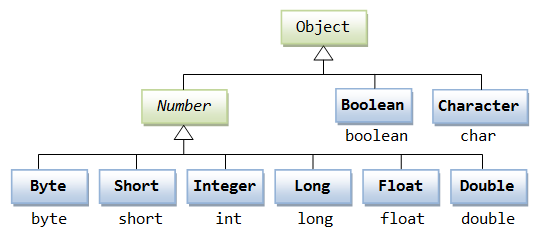# JAVA BEGINNERS TUTORIAL – Dealing With Numbers 1

From time to time I need to have a peek on the basics of any language I know for that reason I am wrting these toturial blogs. any programming language must have to Work with numbers. in java is easy as we use primitive data types such as byte, int, long, double, etc.

### Example:

```int i = 5000;
float gpa = 13.65;

In development, There are reasons to use objects in place of primitives, and the Java platform provides wrapper classes for each of the primitive data types. These classes “wrap” the primitive in an object. All of the numeric wrapper classes (Integer, Long, Byte, Double, Float, Short)  are subclasses of the abstract class Number:This wrapping is taken care of by the compiler, the process is called boxing. So when a primitive is used when an object is required, the compiler boxes the primitive type in its wrapper class. Similarly, the compiler unboxes the object to a primitive as well. The Number is part of the java.lang package.

Here is an example of boxing and unboxing:

```public class Test{

public static void main(String args[]){
Integer x = 5; // boxes int to an Integer object
x =  x + 10;   // unboxes the Integer to a int
System.out.println(x);
}
}```

When x is assigned integer values, the compiler boxes the integer because x is integer objects. Later, x is unboxed so that they can be added as integers.

## Number Methods:

Here is the list of the instance methods that all the subclasses of the Number class implement:

### xxxValue()

The method converts the value of the Number Object that invokes the method to the primitive data type that is returned from the method.

Here is a separate method for each primitive data type:

```byte byteValue()
short shortValue()
int intValue()
long longValue()
float floatValue()
double doubleValue()```

#### Example:

```public class Test{

public static void main(String args[]){
Integer x = 5;
// Returns byte primitive data type
System.out.println( x.byteValue() );

// Returns double primitive data type
System.out.println(x.doubleValue());

// Returns long primitive data type
System.out.println( x.longValue() );
}
}```

### compareTo()

The method compares the Number object that invoked the method to the argument. It is possible to compare Byte, Long, Integer, etc.

However, two different types cannot be compared, both the argument and the Number object invoking the method should be of same type.

`public int compareTo( NumberSubClass referenceName )`
• referenceName — This could be a Byte, Double, Integer, Float, Long or Short.
• If the Integer is equal to the argument then 0 is returned.
• If the Integer is less than the argument then -1 is returned.
• If the Integer is greater than the argument then 1 is returned.

#### Example:

```public class Test{

public static void main(String args[]){
Integer x = 5;
System.out.println(x.compareTo(3));
System.out.println(x.compareTo(5));
System.out.println(x.compareTo(8));
}
}```

### equals()

The method determines whether the Number Object that invokes the method is equal to the argument.

`public boolean equals(Object objectName)`
• The methods returns True if the argument is not null and is an object of the same type and with the same numeric value. There are some extra requirements for Double and Float objects that are described in the Java API documentation.

#### Example:

```public class Test{

public static void main(String args[]){
Integer x = 5;
Integer y = 10;
Integer z =5;
Short a = 5;

System.out.println(x.equals(y));
System.out.println(x.equals(z));
System.out.println(x.equals(a));
}
}```

### valueOf()

Returns the relevant Number Object holding the value of the argument passed. The argument can be a primitive data type, String, etc. This method is a static method.

```static Integer valueOf(int i)
static Integer valueOf(String s)
static Integer valueOf(String s, int radix)```
• i — An int for which Integer representation would be returned.

• valueOf(int i): This returns an Integer object holding the value of the specified primitive.
• s — A String for which Integer representation would be returned.

• valueOf(String s): This returns an Integer object holding the value of the specified string representation.
• radix — This would be used to decide the value of returned Integer based on passed String.

• valueOf(String s, int radix): This returns an Integer object holding the integer value of the specified string representation, parsed with the value of radix.

#### Example:

```public class Test{

public static void main(String args[]){

Integer x =Integer.valueOf(9);
Double c = Double.valueOf(5);
Float a = Float.valueOf("80");

Integer b = Integer.valueOf("444",16);

System.out.println(x);
System.out.println(c);
System.out.println(a);
System.out.println(b);
}
}```

More Methods in thenext blog post